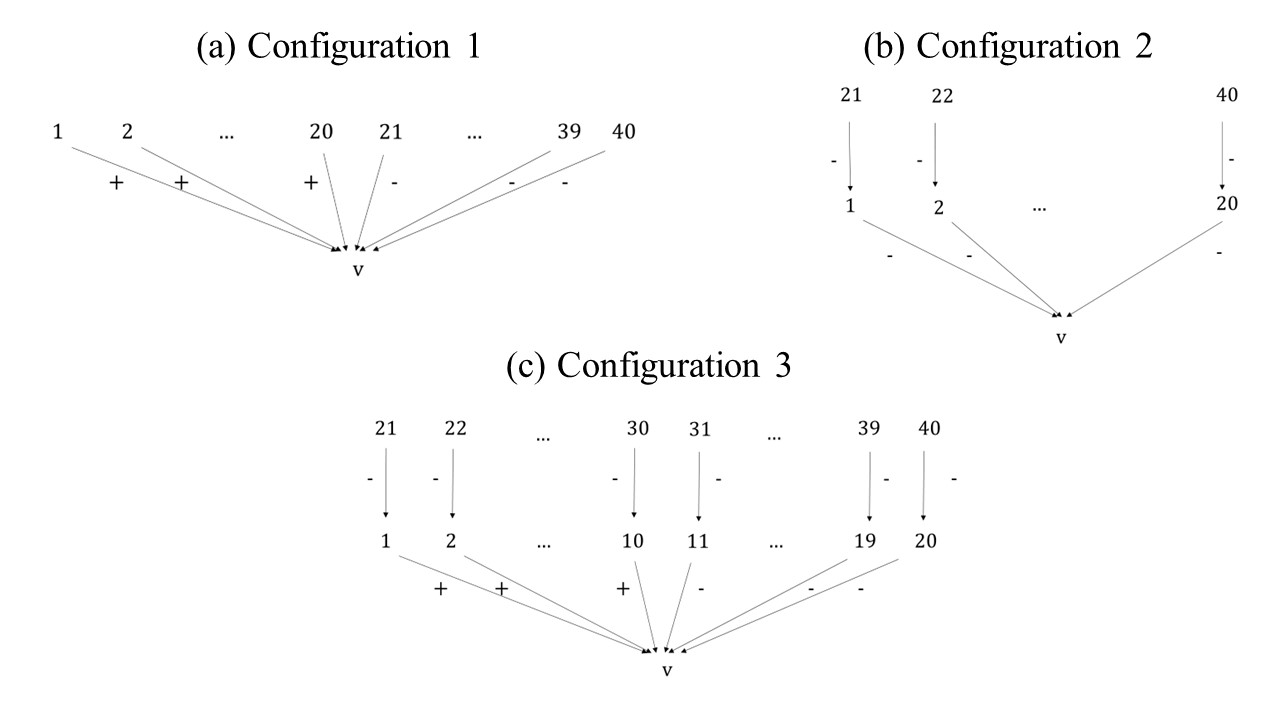### Correction

This new Figure 3 replaces the original Figure 3 in Section 4.1 in Proietti and Chiarella (2023), as Figure 3(a) was, mistakenly, the same as Figure 3(c) in the original version.Figure 3. Three different configurations of the global knowledge base, all with $$Pos(v) = Neg(v) = 20$$. $$s_{\mathbf{G}}(v) = 0.5$$ for (a) and (c) and $$=0.75$$ for (b).

### References

PROIETTI, C., & Chiarella, D. (2023). The Role of Argument Strength and Informational Biases in Polarization and Bi-Polarization Effects. Journal of Artificial Societies and Social Simulation, 26(2), 5. [doi:10.18564/jasss.5062]